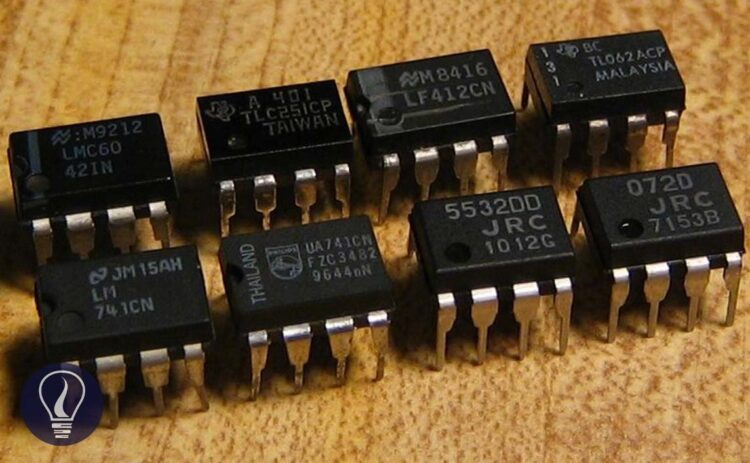More results...

Generic selectors
Exact matches only
Search in title
Search in content
Post Type Selectors

# Op Amp – Operational AmplifierB.Sc (Hons, USJ) (Polymer Science and Technology, Chemistry, Physics)
Categories
Last Updated On : June 24, 2023
Published Date : June 24, 2023

## What is an Operational Amplifier?

An operational amplifier, usually known as an “Op - amp” is an integrated circuit containing several transistors, resistors, a few capacitors, and diodes. Op-amps are used to amplify an external voltage with a very high gain.

For simplicity, op-amp can be shown as follows.

## Voltage Gain

Voltage gain is the ratio between the output voltage and the input voltage. The maximum output voltage is the power supply voltage. this can be obtained by only an ideal op-amp.  In an ideal op -amp the open loop gain is infinite.

Where,

• G = voltage gain
• Vout = output voltage
• Vin =input voltage

## Op-Amp Saturation

There is a maximum output voltage that op-amp can obtain. When this maximum point is exceeded, it is called that the op-amp is saturated. The saturation of the ideal op-amp can be represented as follows.

## Ideal Op-Amp characteristics

In an ideal op -amp following characteristics can be observed.

• Open loop gain is infinite (A = ∞).
• Input impedance is infinite (Rin = ∞).
• Output impedance is zero (Rout = 0).
• The input current is zero.
• Bandwidth is infinite.

### Open loop gain (A)

Open loop gain can be defined as the gain obtained from an op-amp when no feedback is used in the op-amp. When analyzing op–amps we assume that there is no current flow into the input terminal and no current flow out from the output terminal. The open loop gain of an ideal op amp is infinite. It can be obtained as follows.

Input voltage Vin is the voltage difference between the non-inverting and inverting terminals. In an ideal op amp, there is no current flow between inverting and non-inverting terminals. That means there is no voltage difference between the non-inverting and inverting terminals.

### Closed loop gain (G)

The closed-loop gain of an op amp is the gain when feedback is used. Closed loop gain is changed according to the op amp configuration.

Op-Amp Configurations

• Inverting Amplifier
• Non-Inverting Amplifier
• Summing Amplifier
• Differentiator
• Integrator
• Differential Amplifier

### Input impedance (Zin) and output impedance (Zout)

Impedance is the objection of the components in an electrical circuit to the flow of current. If the impedance is generated in a DC system, it is called resistance. Both impedance and resistance values are measured in the same unit: ohms (Ω).

The input impedance In an ideal op amp is infinite. Therefore, there is no current flow between inverting and non-inverting terminals. Output impedance is zero.

### Bandwidth (BW)

The bandwidth of an op amp is the frequency range where the voltage gain is above 70.7%.

### Common mode rejection ratio (CMRR)

Common mode rejection ratio (CMRR) is defined as the ratio between common mode gain to differential mode gain. It is a measurement of the ability to reject noise. If the CMRR is high, that means the op amp has a high ability to reject noise.

Generally, CMRR is expressed in decibels (dB)

Common mode gain is the gain where both inverting and non-inverting terminals of an op amp are applied with the same signal.

## Applications of op-amps

• Audio amplifiers
• Instrumentation amplifiers
• Biomedical systems,
• Analytical Instrument Analog sensors
• Medical equipment
• Power amplifiers
• Analog computers
• Combination of integrators
• Differentiators
• Summing amplifiers
• Multipliers
• Multi-vibrators and Oscillators## References and Attributes

### Figures:

The cover image was designed using an image by Omegatron, licensed under CC BY-SA 3.0, via Wikimedia Commons

Figure 01: Contains an image by Omegatron, licensed under CC0, via Wikimedia Commons

Figure 02: Contains an image by TedPavlic, licensed under CC BY-SA 3.0, via Wikimedia Commons

Figure 03: Contains an image by Omegatron, licensed under CC BY-SA 3.0, via Wikimedia Commons

Figure 05: Contains an image by Inductiveload, licensed under the Public domain, via Wikimedia Commons

Figure 06: Contains an image by Ong saluri, licensed under CC BY-SA 3.0, via Wikimedia Commons